# Analytical reasoning test-2

1)

In each of the following question, find the minimum number of triangles in the given figure.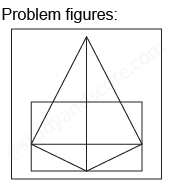2)

In each of the following question, find the minimum number of triangles in the given figure.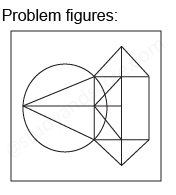3)

In each of the following question, find the minimum number of triangles in the given figure.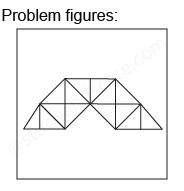4)

In each of the following question, find the minimum number of triangles in the given figure.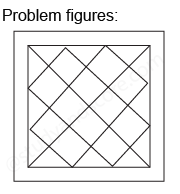5)

What is the number of straight lines and number of triangles in the given figure?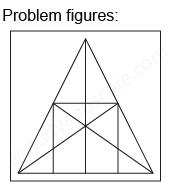--Share with your friends --